# Write The Polynomial As The Product Of Linear Factors And List All The Zeros Of The Function. H(X) = X^3 – 3x^2 + 4x – 2

We thoroughly check each answer to a question to provide you with the most correct answers. Found a mistake? Let us know about it through the REPORT button at the bottom of the page.

Write the polynomial as the product of linear factors and list all the zeros of the function. h(x) = x^3 – 3x^2 + 4x – 2

The constant term of the given function is -2 and its factors are +-2 and +-1.

The leading coefficient of the given function is 1 and its factors are +-1.

By dividing factors of the constant term by factors of the leading coefficient, we get the possible rational zeros. Therefore, possible rational zeros are +-2 and +-1.

Let us use the synthetic division to show that x = 1 is a zero of x^3 – 3x^2 + 4x – 2: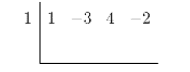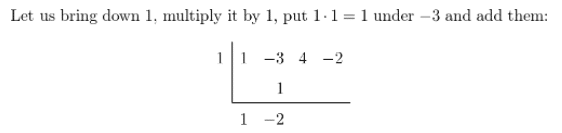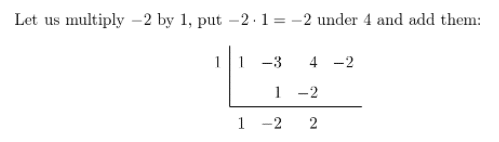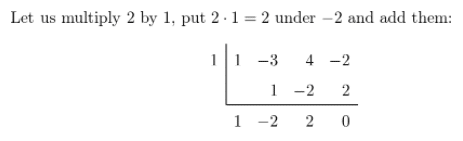We got x^2 – 2x + 2 and the remainder of 0. Therefore x = 1 is a zero of the given function.

Let us use the quadratic formula to find the zeros of x^2 – 2x + 2. We have a = 1, b = -2 and c = 2.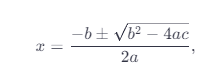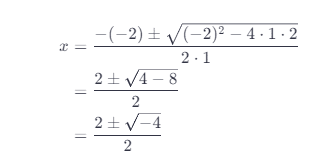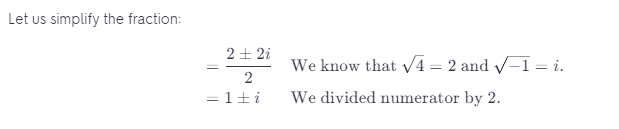THe factored function is h(x) = (x-1)(x-1-i)(x-1+i).

Zeros of the given function are x =1 and x = 1 +-i.

Zeros of the function h(x) = (x-1)(x-1-i)(x-1+i) are x = 1 and x = 1+-i.